Question

Find the equivalent resistance between points A and B in the drawing.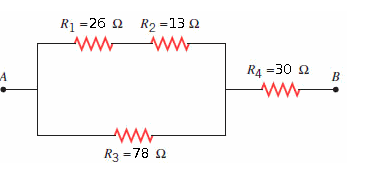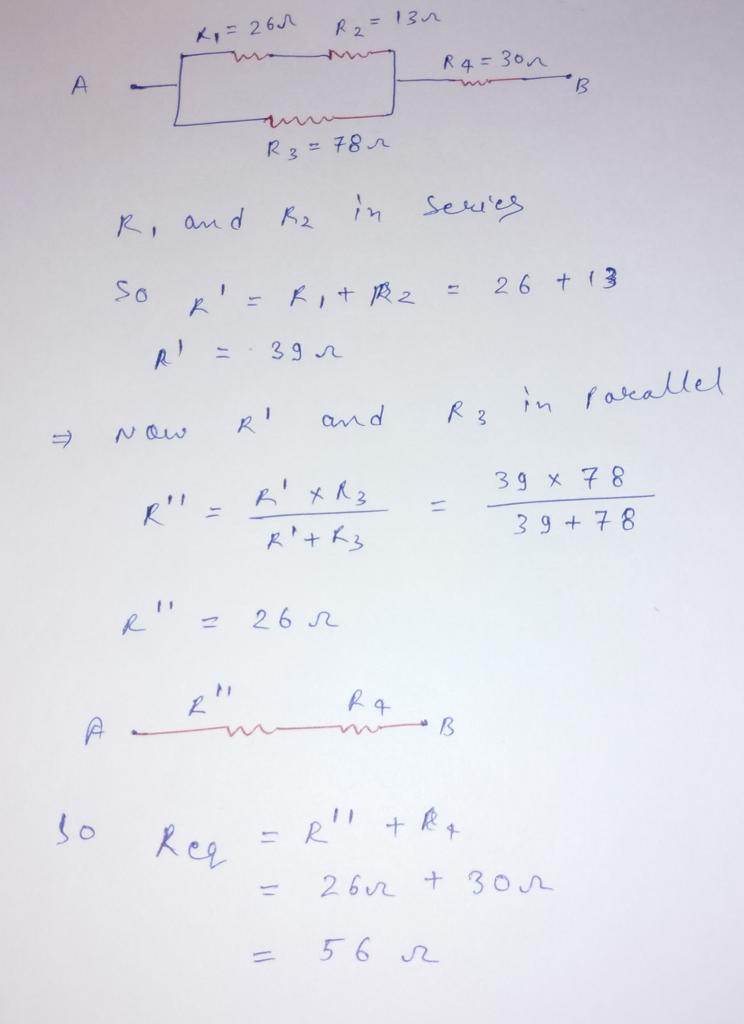#### Earn Coins

Coins can be redeemed for fabulous gifts.

Similar Homework Help Questions
• ### a) Find the equivalent resistance between the points A and C in the wing. (b) If...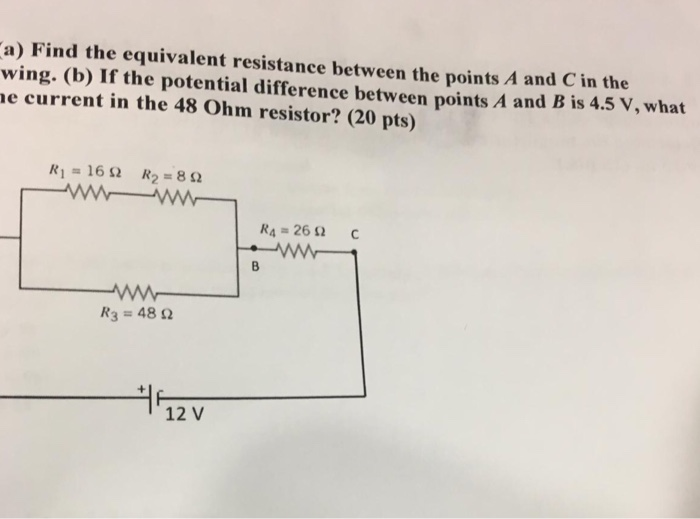a) Find the equivalent resistance between the points A and C in the wing. (b) If the potential difference between points A and B is 4.5 V, what e current in the 48 Ohm resistor? (20 pts) Ri=16Ω R2 = 8 Ω R4-26 Ω R3 = 48 Ω 12 V

• ### Find the equivalent resistance between points A and B in the drawing. Find the equivalent resistance...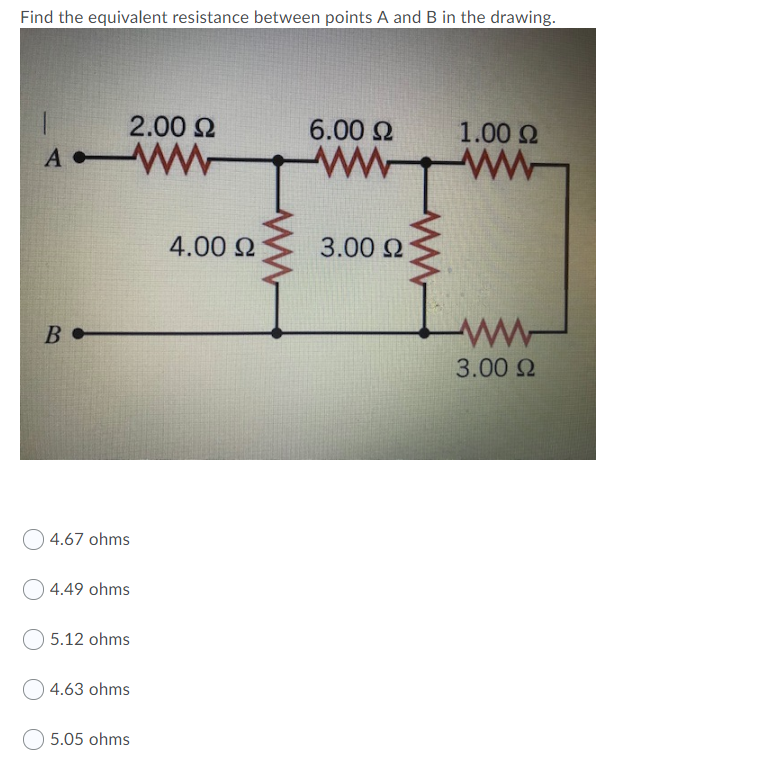Find the equivalent resistance between points A and B in the drawing. Find the equivalent resistance between points A and B in the drawing. 2.00 Ω 6.00 Ω 1.00 Ω A 4.00 Ω 3.00 Ω B - ΑΛΛ- 3.00 Ω 4.67 ohms Ο4.49 ohms 5.12 ohms 4.63 ohms 5.05 ohms

• ### Find the equivalent resistance between points A and B in the drawing 2.00 Ω 6.00 Ω...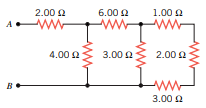Find the equivalent resistance between points A and B in the drawing 2.00 Ω 6.00 Ω 1.00 Ω wongan 4.00 Ω 3.00Ω 2.00 Ω B 3.00 Ω

• ### Find the equivalent resistance between points A and B in the drawing 2.90 Ω 6.50 Ω...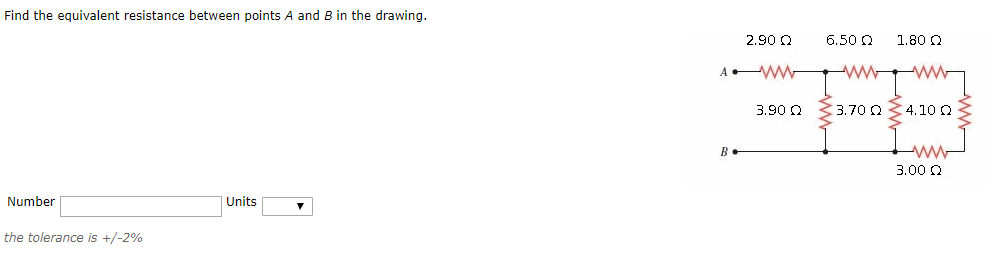Find the equivalent resistance between points A and B in the drawing 2.90 Ω 6.50 Ω 1.80 Ω 3.90 Ω 3.70 Ω 4.10 Ω 3.00 Ω Number Units the tolerance is +/-296

• ### 4. Find the equivalent resistance between points A and B in the drawing (R1 = 4.0...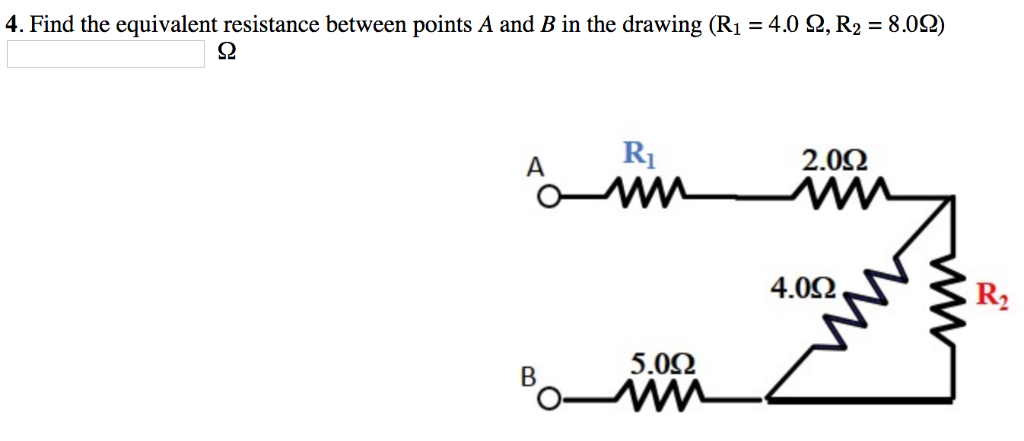4. Find the equivalent resistance between points A and B in the drawing (R1 = 4.0 Ω, R2 = 8.0Ω) S2 2.0Ω 4.0Ω R2 5.0Ω

• ### Find the equivalent resistance between points A and B in the drawing. 3.0 Ω 4.0 2...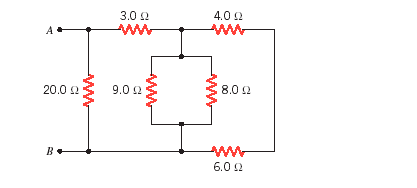Find the equivalent resistance between points A and B in the drawing. 3.0 Ω 4.0 2 8.0 Ω 20012 9.0 12 6.0 Ω

• ### Find the equivalent resistance between points A and B shown in the figure(Figure 1). Consider Ri...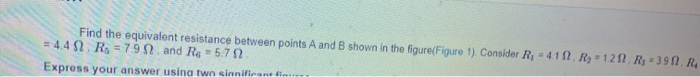Find the equivalent resistance between points A and B shown in the figure(Figure 1). Consider Ri - 411.R, 121. Ry - 39 1. R4 = 4.41 Rs = 791 and Rs = 5.722 Express your answer using two sinnificant in A w R, w RA R, R, B RO

• ### 9. Determine the equivalent resistance between the points A and B for the group of resistors...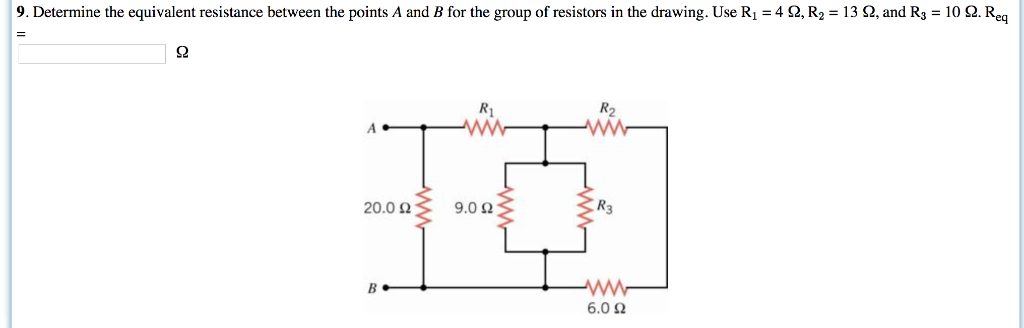9. Determine the equivalent resistance between the points A and B for the group of resistors in the drawing. Use R1-4 Ω, R2 = 13 Ω and R3 = 10 Ω Re Ri R2 20.0 Ω 9.0 Ω R3 6.0 Ω

• ### Question 3. (10 points) Four resistors are connected as shown in Figure 28.9a Find the equivalent resistance between a and c. R1-16 and R4-12 Ω R2-13 R3-4 (a) (b) What is the current in each resistor...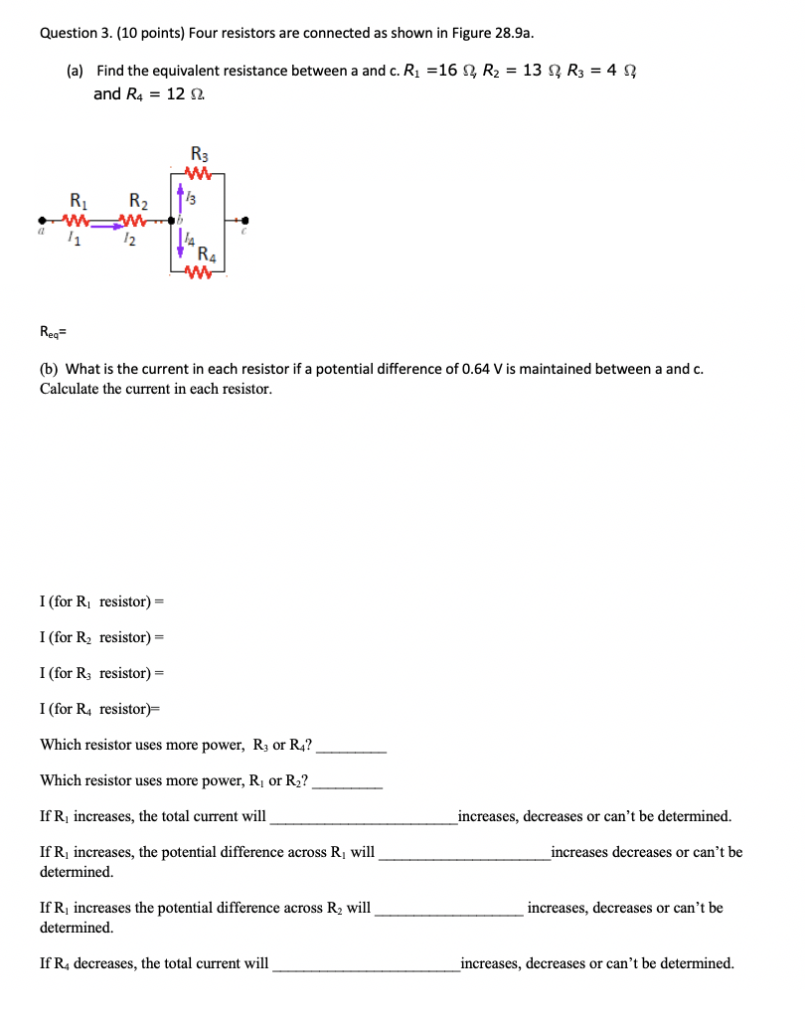Question 3. (10 points) Four resistors are connected as shown in Figure 28.9a Find the equivalent resistance between a and c. R1-16 and R4-12 Ω R2-13 R3-4 (a) (b) What is the current in each resistor if a potential difference of 0.64 V is maintained between a and c. Calculate the current in each resistor I (for Ri resistor)- I (for R2 resistor)- I (for R3 resistor) I (for R4 resistor)- Which resistor uses more power, R or R4? Which...

• ### Question 2. (15 points) Four resistors are connected as shown in Figure 28.9a (a) Find the equivalent resistance between a and c. R1-16 Ω, R2-139, R3-49, and R4-12 Ω Rs (b) What is the current in eac...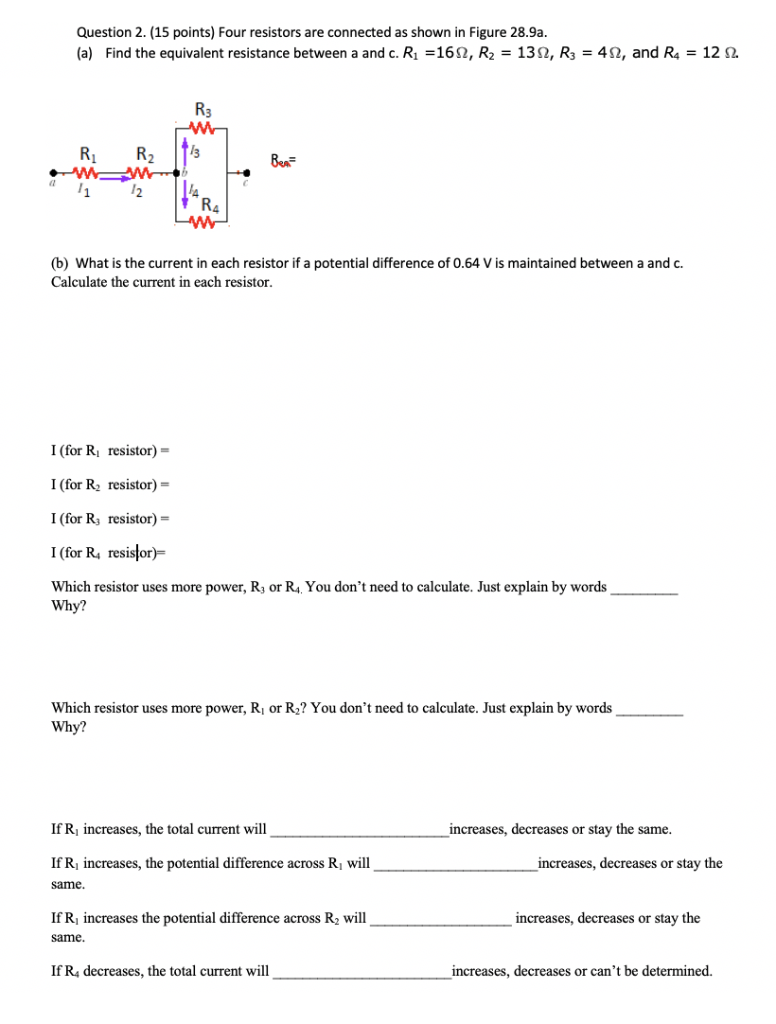Question 2. (15 points) Four resistors are connected as shown in Figure 28.9a (a) Find the equivalent resistance between a and c. R1-16 Ω, R2-139, R3-49, and R4-12 Ω Rs (b) What is the current in each resistor if a potential difference of 0.64 V is maintained between a and. Calculate the current in each resistor I (for Ri resistor)- I (for R resistor) I (for R, resistor)- I (for R resistor Which resistor uses more power, R or R,....